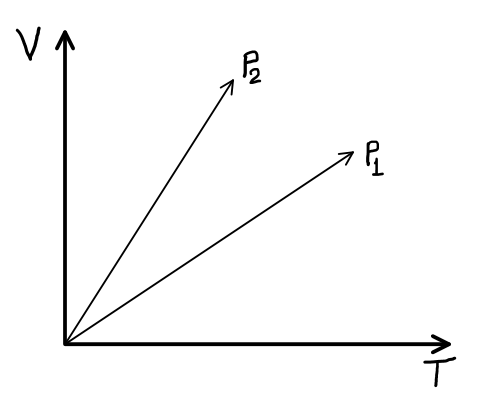Doubtnut App - Get 50 Lakh+ video solutions better than GoogleInstall Now

# Figure shows the volume versus temperature graph for the same mass of a gas (assumed ideal) corresponding to two different pressure P1andP2. ThenA

P1>P2

B

P1<P2

C

P1=P2

D

The information is insufficient

Text Solution
Verified by Experts

## Since the graph is a straight line. so, V=mT where m is the slope, =(nRT)/P [from equation of state] or m∝(1/P) or, m2>m1∴P2<P1.